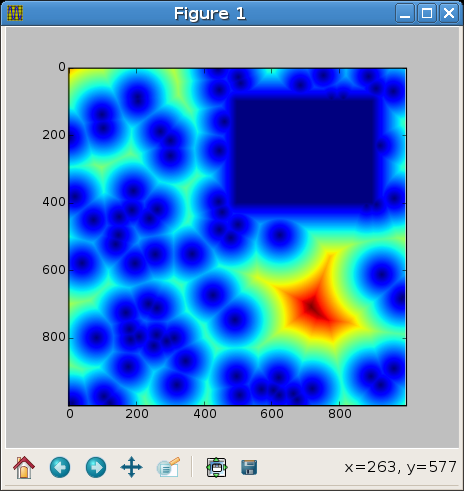Euclidean Distance Transform in Python

This may be useful to someone.

Algorithmic complexity doesn't seem bad, but no guarantees. To use, pass distance_transform a 2D boolean numpy array. Psyco helps. So would rewriting it in C.

```def _upscan(f):
for i, fi in enumerate(f):
if fi == numpy.inf: continue
for j in xrange(1,i+1):
x = fi+j*j
if f[i-j] < x: break
f[i-j] = x

def distance_transform(bitmap):
f = numpy.where(bitmap, 0.0, numpy.inf)
for i in xrange(f.shape):
_upscan(f[i,:])
_upscan(f[i,::-1])
for i in xrange(f.shape):
_upscan(f[:,i])
_upscan(f[::-1,i])
numpy.sqrt(f,f)
return f
```[æ]